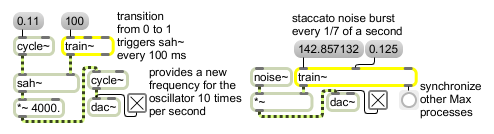# train~

Pulse train generator

## Description

train~ generates a pulse signal whose period is specifiable in terms of milliseconds (see <~ for a method of making a pulse wave at a specified frequency). It also sends out a bang when going from 0 to 1, so it can be used as a metronome with a floating-point interval. The signal output can be used as a control input for sah~ as shown in the example below, or at very small intervals, it is audible.

## Arguments

Name Type Opt Description
inter-pulse-interval pulse-width and phase float or int opt Initial values for inter-pulse interval in milliseconds (default 1000), pulse width (default 0.5), and phase (default 0). If signal objects are connected to any of the train~ object's inlets, the corresponding initial argument value is ignored.

## Messages

 int parameter-settings [int] Numbers can be used instead of signal objects to control period, pulse width, and phase. If a signal is also connected to the inlet, float and int messages are ignored. float parameter-settings [float] Numbers can be used instead of signal objects to control period, pulse width, and phase. If a signal is also connected to the inlet, float and int messages are ignored. signal In left inlet: Specifies the period (time interval between pulse cycles), in milliseconds, of a pulse train sent out the left outlet. In middle inlet: Controls the pulse width or duty cycle. The signal values represent a fraction of the pulse interval that will be devoted to the "on" part of the pulse (signal value of 1). A value of 0 has the smallest "on" pulse size (usually a single sample), while a value of 1 has the largest (usually the entire interval except a single sample). A value of 0.5 makes a pulse with half the time at 1 and half the time at 0. In right inlet: Sets the phase of the onset of the "on" portion of the pulse. A value of 0 places the "on" portion at the beginning of the interval, while other values (up to 1, which is the same as 0) delay the "on" portion by a fraction of the total inter-pulse interval.

## Output

bang: Out right outlet: When the "on" portion of the pulse begins, a bang is sent out the right outlet. Using this outlet, you can use train~ as a signal-synchronized metronome with an interval specifiable as a floating-point (or signal) value. However, there is an unpredictable delay between the "on" portion of the pulse and the actual output of the bang message, which depends in part on the current Max scheduler interval. The delay is guaranteed to be a millisecond or less if the scheduler interval is set to 1 millisecond.
signal: Out left outlet: A pulse (square) wave train having the specified interval, width, and phase.

## ExamplesProvide an accurate pulse for rhythmic changes in signal